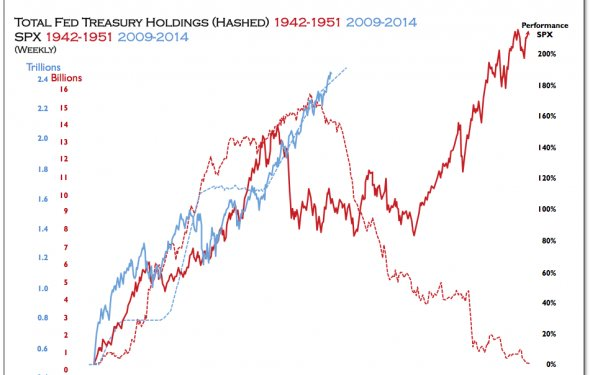# Bond Market and interest rates

Understanding Interest Rates, Inflation And Bonds
June 28, 2017 – 07:21 amOwnership of a bond is the ownership of a stream of future cash payments. Those cash payments are usually made in the form of periodic interest payments and the return of principal when the bond matures. In the absence of credit risk (the risk of default), the value of that stream of future cash payments is simply a function of your required return based on your inflation expectations. If that sounds a little confusing and technical, don't worry, this article will break down bond pricing, define the term "bond yield" and demonstrate how inflation expectations and interest rates determine the value of a bond.

Measures of Risk

There are two primary risks that must be assessed when investing in bonds: interest rate risk and credit risk. Though our focus is on how interest rates affect bond pricing, otherwise known as interest rate risk, it's also important that a bond investor be aware of credit risk. Interest rate risk is the risk of changes in a bond's price due to changes in prevailing interest rates. Changes in short-term versus long-term interest rates can affect various bonds in different ways, which we'll soon discuss. Credit risk, meanwhile, is the risk that the issuer of a bond will not make scheduled interest and/or principal payments. The probability of a negative credit event or default affects a bond's price - the higher the risk of a negative credit event occurring, the higher the interest rate investors will demand for assuming that risk.

Bonds issued by the United States Treasury to fund the operation of the U.S. government are known as U.S. Treasury bonds. Depending on the time until maturity, they are called bills, notes or bonds. Investors consider U.S. Treasury bonds to be free of default risk. In other words, investors believe there is no chance that the U.S government will default on interest and principal payments on the bonds it issues. For the remainder of this article, we will use U.S. Treasury bonds in our examples, thereby eliminating credit risk from the discussion.

Calculation of a Bond's Yield and Price

To understand how interest rates affect a bond's price, you must understand the concept of yield. While there are several different types of yield calculations, for the purposes of this article, we will use the yield-to-maturity (YTM) calculation. A bond's YTM is simply the discount rate that can be used to make the present value of all of a bond's cash flows equal to its price. In other words, a bond's price is the sum of the present value of each cash flow where the present value of each cash flow is calculated using the same discount factor. This discount factor is the yield. When a bond's yield rises, by definition, its price falls, and when a bond's yield falls, by definition, its price increases.

A Bond's Relative Yield

The maturity or term of a bond largely affects its yield. To understand this statement, you must understand what is known as the yield curve. The yield curve represents the YTM of a class of bonds (in this case, U.S. Treasury bonds). In most interest rate environments, the longer the term to maturity, the higher the yield will be. This should make intuitive sense because the longer the period of time before a cash flow is received, the more chance there is that the required discount rate (or yield) will move higher.

Inflation Expectations Determine Investors Yield Requirements

Inflation is a bond's worst enemy. Inflation erodes the purchasing power of a bond's future cash flows. Put simply, the higher the current rate of inflation and the higher the (expected) future rates of inflation, the higher the yields will rise across the yield curve, as investors will demand this higher yield to compensate for inflation risk.

Source: www.investopedia.com
Related Posts